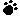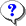Note: You can hover cursor toto find the correct answer.0  (0%)24 Sec

1.A project is estimated to cost \$ 50,000 with a timeline of 50 days. After 25 days, the project manager finds that 50% of the project is complete and Actual costs are \$ 50,000. What is the Cost Performance Index (CPI) ?

A) The CPI is 1
B) The CPI is 1.5
C) The CPI is 2
D) The CPI is 0.5Your answer=, Correct answer=, Work Sheet:

2.The Cost Performance Baseline is a time-phased budget and is used as a basis to measure, monitor, and control overall cost performance of the project. It is usually displayed in the form of:

A) An S-curve
B) An inverted S curve.
C) Pie-chart
D) A Z curveYour answer=, Correct answer=, Work Sheet:

3.As a project manager, you periodically do project performance reviews to compare cost performance over time, schedule activities or work packages over-running and under-running budget, milestones due, and milestones met. Which of the following is not a valid technique that you would use for performance-reporting?

A) Trend Analysis
B) Earned Value Performance
C) Variance Analysis
D) Integration AnalysisYour answer=, Correct answer=, Work Sheet:

4.An estimating technique that uses a statistical relationship between historical data and other variables (for example, square footage in construction, lines of code in software development) is known as:

A) Parametric Estimating
B) Analogous Estimating
C) Bottom-up Estimating
D) Historical AnalysisYour answer=, Correct answer=, Work Sheet:

5.A variance threshold for costs or other indicators to indicate the agreed amount of variation allowed is called:

A) Variance limits
B) Control threshold
C) Cost limit
D) Acceptable thresholdYour answer=, Correct answer=, Work Sheet:

 Click Turn Right Ahead sign for next test